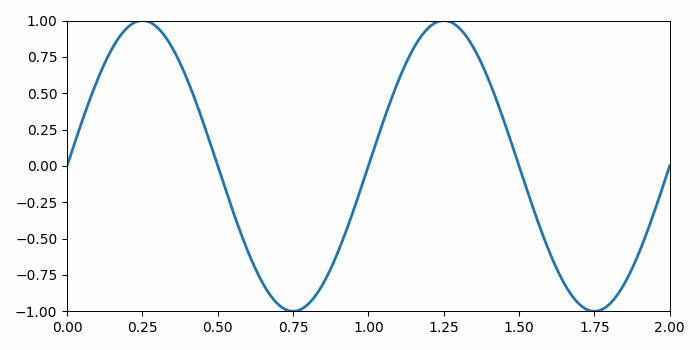# Embedding a matplotlib animation into a tkinter frame

MatplotlibPythonData Visualization

#### Python Data Science basics with Numpy, Pandas and Matplotlib

Most Popular

63 Lectures 6 hours

#### Data Visualization using MatPlotLib & Seaborn

11 Lectures 4 hours

#### MatPlotLib with Python

9 Lectures 2.5 hours

To embed a matplotlib animation into a tkinter frame, we can take the following steps

## Steps

• Set the figure size and adjust the padding between and around the subplots.

• Create a Toplevel widget of Tk which represents mostly the main window of an application

• Set the title of this widget.

• Add an axes to the current figure and make it the current axes.

• Create a new figure or activate an existing figure.

• Add an 'ax' to the figure as part of a subplot arrangement.

• Make a dummy line plot with linewidth=2.

• Create the canvas the figure renders into.

• Create the figure canvas on which to operate.

• Create a keypress event to quit the tkinter winter.

• Create an animation by repeatedly calling a function *animate*.

• To display the figure, use Show() method.

## Example

import tkinter
from matplotlib.backends.backend_tkagg import (
from matplotlib.backend_bases import key_press_handler
from matplotlib import pyplot as plt, animation
import numpy as np

plt.rcParams["figure.figsize"] = [7.00, 3.50]
plt.rcParams["figure.autolayout"] = True

root = tkinter.Tk()
root.wm_title("Embedding in Tk")

plt.axes(xlim=(0, 2), ylim=(-2, 2))
fig = plt.Figure(dpi=100)
ax = fig.add_subplot(xlim=(0, 2), ylim=(-1, 1))
line, = ax.plot([], [], lw=2)

canvas = FigureCanvasTkAgg(fig, master=root)
canvas.draw()

toolbar = NavigationToolbar2Tk(canvas, root, pack_toolbar=False)
toolbar.update()

canvas.mpl_connect(
"key_press_event", lambda event: print(f"you pressed {event.key}"))
canvas.mpl_connect("key_press_event", key_press_handler)

button = tkinter.Button(master=root, text="Quit", command=root.quit)
button.pack(side=tkinter.BOTTOM)

toolbar.pack(side=tkinter.BOTTOM, fill=tkinter.X)
canvas.get_tk_widget().pack(side=tkinter.TOP, fill=tkinter.BOTH, expand=1)

def init():
line.set_data([], [])
return line,

def animate(i):
x = np.linspace(0, 2, 1000)
y = np.sin(2 * np.pi * (x - 0.01 * i))
line.set_data(x, y)
return line,

anim = animation.FuncAnimation(fig, animate, init_func=init,frames=200, interval=20, blit=True)

tkinter.mainloop()

## Output

It will produce the following output −